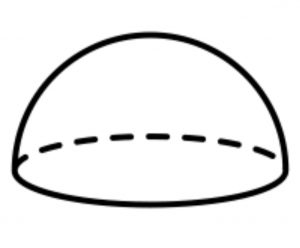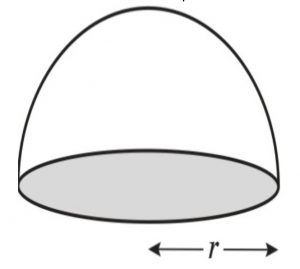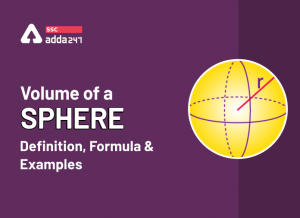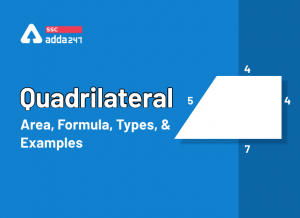Latest SSC jobs   »   Hemisphere   »   Hemisphere

# Hemisphere Definition, Volume, Formula, Examples and Total Surface Area

## Hemisphere Definition

Hemisphere: Generally, we observe various two-dimensional and three-dimensional shapes having their specific properties. The three-dimensional objects cannot be shown on paper because it has all three axes. The sphere is made by rotating a 2-dimensional circle, it is a three-dimensional object. But the hemisphere is simply half of the sphere. When a sphere is cut into two equal parts at the center then the hemisphere is formed. The best example of the hemisphere is, Bowl, Earth has exactly two hemispheres namely the southern hemisphere and the northern hemisphere.## Hemisphere Volume

The volume of the hemisphere is the space occupied by the hemispherical object. The hemisphere has a larger size occupies more volume and the hemisphere having a lesser size occupies less volume. A hemisphere is half of the sphere It has one flat circular base and one curved surface. The volume of the hemisphere is represented in cubic units.Volume of hemisphere = (2/3)πr^3

where π is a constant term having a value of 3.14 and r is the radius of the circle

## Hemisphere Total Surface Area

The surface area of the hemisphere is defined as the outer surface of the hemisphere including the area including the base called a total surface area. There are two surface areas on the hemisphere namely curved surface area and total surface area which are discussed below:

Curved Surface Area – It is defined as the area of the outer surface of the hemisphere. It includes only the surface of the hemisphere.

The curved surface area of the Cylinder = ½ of the total surface area

= ½ × 4πr^2

= 2πr^2

Total Surface Area – The total surface area of the hemisphere is the area that includes the outer surface and base. It is also defined as the sum of curved surface area and the base area of the hemisphere.

The total surface area of the hemisphere = 3πr^2

## Hemisphere Formula Volume

The volume of a hemisphere is the space occupied by a hemispherical object. Hemisphere is exactly half of the sphere. The volume of a hemisphere is expressed in cubic units i.e. m^3, cm^3, and mm^3. The volume of the hemisphere includes the volume of the curve and base. The formula for the volume of a hemisphere is shown below:

The volume of hemisphere = ⅔πr^3

where, π = 3.14 (constant value)

r = radius of the circle

## Hemisphere: Solved Examples

Que.1 If the diameter of the hemisphere is 8cm. Then find the volume of the hemisphere?

Solution: Given that

Diameter of hemisphere d = 8cm

We know that, d = 2r

then, r = 4cm

The volume of hemisphere = 2/3πr^3

= 2/3×3.14×(4)^3

= 133.97 = 134 nearly

Que.2 A hemisphere has a radius of 4.5cm. Calculate the curved surface area of the hemisphere?

Solution: Given data

r = 4.5m

Curve surface area =?

CSA = 2πr^2

= 2×3.14×4.5×4.5

= 127.17m^2

Que.3 A hemisphere having a radius 3of cm. Find the total surface area of the hemisphere?

Ans – Given r = 3cm

TSA = 3πr^2

= 3×3.14×3×3

= 84.78

Que.4 Find the surface area of the hemisphere if the radius of the hemisphere is 2cm.

Solution: Given r = 2cm

The surface area of the hemisphere = 2πr^2

= 2×3.14×2×2

= 25.12

Que.5 The radius of the hemisphere is 3cm then find the volume of the hemisphere?

Solution: Given r = 8cm

The volume of hemisphere = 3πr^3

= 3×3.14×3×3×3

= 254.34cm^3

Que.6 What is the total surface area of a solid hemispherical object of radius 7cm?

Solution: We know that the total surface area of a solid hemisphere is calculated as A = 3πr^2

the radius of the object is 7cm

then the total surface area of a solid object is

9 = 3×3.14×7×7

= 462cm^2

Que.7 A hemispherical bowl has an inner radius of14cm. How much amount of water it can contain?

Solution: We know that the volume of a hemisphere is calculated as

V = 2/3πr^3

the radius of the bowl = 14cm

So the volume of the bowl = 2/3×3.14×14×14

= 5750cm^3

Que.8 Find the radius of the hemisphere when its total surface area is 462squareunits.

Solution: Given, that the total surface area of the object = 462sq.units

the total surface area of the object is calculated as A = 3πr^2

462 = 3×π×r^2

r^2 = 49

r = 7 units

Que.9 Find the volume of the hemisphere when its area of the circular base is 154 sq. units.

Solution: Given, the area of base = 154 sq. units

The formula for the area of the circular base is πr^2

πr^2 = 154

r^2 = 154/π

r^2 = 49

r = 7 units

The volume of hemisphere = 2/3πr^3

= 2/3×3.14×7×7×7

= 718.67 cubic units

Que.10 Find the radius of the hemispherical bowl if its area of the base is 144cm. Where π = 22/7

Solution: We know that the area of the base of the hemisphere is πr^2

144 = 22/7

r^2 = 144×7/22

r^2 = 45.81

r = √45.81

r = 6.76 units

### Hemisphere – FAQs

Que.1 Define the term hemisphere?

Ans – A hemisphere is formed by dividing the sphere into two equal parts. It is the parts of a sphere divided at the plane at the center of the sphere. An example of the hemisphere is earth, bowl, cup, ice cream scoop, hat, etc.

Que.2 What is the volume of the hemisphere?

Ans – The volume of a hemisphere is the space occupied by the hemisphere. It is expressed in cubic units. The formula for the volume of a hemisphere is 3πr^3.

Que.3 Define the term curved surface area of the hemisphere?

Ans – The curved surface area is the outer area of the hemisphere. The formula of the curved surface area of the hemisphere is 2πr^2.

Que.4 What is the total surface area of the hemisphere?

Ans – The total surface area is the sum of curved surface area and base area. The formula of the total surface area is 3πr^2.

#### Congratulations!General Awareness & Science Capsule PDF•Elementary Statistics - Problems, Questi...
•Histogram Definition, Graph, Chart, Form...
•What is Rectangle? Definition, Area, For...
•Volume of a Sphere
•Quadrilateral Area, Formula, Types, Prop...